Reach Us+44-1522-440391
A Prime Radical of Weakly Artinian and#937;-Groups with Finite Condition is Locally Nilpotent | OMICS International
Journal of Generalized Lie Theory and Applications
All submissions of the EM system will be redirected to Online Manuscript Submission System. Authors are requested to submit articles directly to Online Manuscript Submission System of respective journal.

# A Prime Radical of Weakly Artinian Ω-Groups with Finite Condition is Locally Nilpotent

Blagovisnaya A, Pikhtilkov S* and Pikhtilkova O

Faculty of Mathematics, Orenburg State University, 13 Pobedy Avenue, 460018, Orenburg, Russia

Corresponding Author:
Pikhtilkov S
Faculty of Mathematics, Orenburg State University
13 Pobedy Avenue, 460018, Orenburg, Russia
Tel: +73532776770
E-mail: [email protected]

Received date: date: October 01, 2015; Accepted date: November 21, 2015; Published date: November 28, 2015

Citation: Blagovisnaya A, Pikhtilkov S, Pikhtilkova O (2015) A Prime Radical of Weakly Artinian Ω-Groups with Finite Condition is Locally Nilpotent. J Generalized Lie Theory Appl 9:237. doi:10.4172/1736-4337.1000237

Copyright: © 2015 Blagovisnaya A, et al. This is an open-access article distributed under the terms of the Creative Commons Attribution License, which permits unrestricted use, distribution, and reproduction in any medium, provided the original author and source are credited.

Visit for more related articles at Journal of Generalized Lie Theory and Applications

#### Abstract

First we discuss the background of the issue to Lie algebras. We also show the relationship of our problem and A.V. Mikhalev’s problem. The main result is proved for Lie algebras. We then consider the Ω-group".

#### Keywords

Weakly artinian lie algebra; Locally nilpotent lie algebra; Prime radical of a lie algebra; Ω-group

#### Introduction

First we discuss the background of the work for Lie algebras. We also show the relationship of our problem and Mikhalev’s problem. The main result is proved for Lie algebras. We then consider the Ω-group.

Definition 1. A Lie algebra L is said to be prime if [U,V] = 0 implies U = 0 or V = 0 for any ideals U and U of L.

Definition 2. We say that the ideal P of a Lie algebra P is prime if the factor algebra L / P is prime.

Definition 3. The intersection of all prime ideals is called the prime radical P(L) of a Lie algebra L.

The reader may consult the paper of Balaba , about theory of the prime radical of Lie algebras.

Definition 4. A Lie algebra is said to be weakly artinian if the Lie algebra satisfies the descending chain condition on ideals.

In 2001, Mikhalev has formulated the problem at the seminar “Rings and modules” in Moscow State University: does there exist weakly artinian Lie algebra prime radical of which is not solvable?

In studies of Pikhtilkov , proved that the prime radical of special weakly artinian Lie algebra is solvable. It was shown in literature of Sergey , that prime radical of weakly artinian locally nilpotent Lie algebras is solvable.

Weakened Mikhalev’s problem is solved in framework of Mescherina . It is proved that the prime radical of Lie algebra is solvable if the Lie algebra satisfies the descending chain condition for inner ideals or subalgebras.

It is known that the prime radical of Lie algebra is weakly solvable, it may not be locally solvable .

Now we prove the following theorem.

Theorem 1. Let L be a weakly artinian Lie algebra and P = P(L) be its prime radical. Then P(L) is locally nilpotent.

Proof. To prove the theorem we need to consider the representation of a prime radical of the Lie algebra as the lower weakly solvable radical. This representation was considered in studies of Mescherina .

Let σ (L) be any nonzero abelian ideal of P(L). The ideal σ (L) is contained in the prime radical. Therefore this ideal is exists.

This ideal is contained in any nonzero solvable ideal of prime radical P(L). If P(L) ≠ 0, then abelian ideal of prime radical P(L) exists according to the construction of the lower weakly solvable radical . If P(L) = 0, there is nothing to prove. It was proved in literature of Jacobson , that any nonzero solvable ideal contains a nonzero abelian ideal.

Further we define an ideal τ (α) ⊂ P(L) for each ordinal number α using transfinite induction. We get the following.

1. τ (0) = 0.

2. Suppose that for all α < β ideals τ (α) is defined. Then we define τ (β) as follows.

a) τ (β) is an ideal of the algebra L such that τ (β) /τ (γ) = σ (L / τ (γ)) if β = γ + 1 is not a limit ordinal.

b) If β is a limit ordinal, then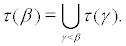For reasons of the cardinality we have τ (β) = τ (β +1) for some β. Then τ (β) = P(L).

Now we construct another representation of prime radical using nilpotent ideals.

Let σ (L) be a sum of all nonzero abelian ideals of P(L). From weakly artinianess of Lie algebra L, it follows that those abelian ideals are finite.

In the studies of Jacobson  it was shown that the sum of nilpotent ideals of Lie algebra is nilpotent. Therefore, the ideal σ (L) is nilpotent.

As above we define the ideal of σ (α) ⊂ P(L) for each ordinal number α using transfinite induction. We get the following.

1. σ (0) = 0.

2. Suppose that σ (α) is defined for all α < β. Then we define α (β) as follows.

a) α (β) is an ideal of the algebra L such that σ (β) /σ (γ) = σ (L / σ (γ)) if β = γ + 1 is not a limit ordinal.

b) If β is a limit ordinal, thenFor reasons of the cardinality we have σ (β) = σ (β +1) for some β. Then σ (β) = P(L).

Denote by N(σ (L)) the degree of nilpotency of the ideal σ (L).

Let be X ⊂ P(L) is a nonempty finite set. We shall prove that everything [xi1, xi2, ....., xir], xik ∈ X with the left arrangement of brackets is equal to 0 for some natural r.

For each x ∈ X we denote by α (x) is an ordinal α such that x∈σ (α) \ σ (α −1) is defined, and α, if x∈σ (α) and α − 1 is not defined.

Denote by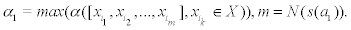Consider all the products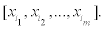Those products satisfy the condition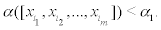By definition, put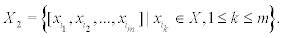Denote by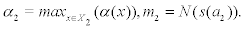From the above it follows that α2 < α1.

Further by definition, put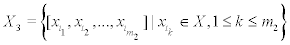We shall get a sequence of sets X1, X2,… and a decreasing sequence of ordinal numbers α1 > α2 >…, which cannot be infinite.

Therefore, for some k all elements of Xk are zero.

Hence the Lie algebra generated by set X is nilpotent. Then the prime radical P(L) is locally nilpotent.

According Theorem we get restrictions for constructing the counterexample to Mikhalev’s problem.

Let L be a weakly artinian Lie algebra. Let P = P(L) denote prime radical of L.

Consider the derived series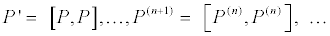Then P ⊃ P′ ⊃ P′′⊃ … ⊃ P(n) ⊃ … .

As the Lie algebra L is a weakly artinian then the decreasing chain of its ideals is stabilized, i.e., R = P(n+1) = P(n) for some natural n. Hence [R, R] = R.

There is a desire to find weakly artinian algebra R which is not solvable and satisfying the following conditions:

1) [R, R] = R;

2) P(R) = R.

It was proved in literature of Pikhtilkov , that the prime radical of the locally nilpotent weakly artinian Lie algebra is solvable.

From theorem 1 it follows that the Lie algebra R is locally nilpotent. We proved that the Lie algebra R is solvable.

Consequently, the Lie algebra L, that is a counterexample to Mikhalev’s problem, must satisfy condition P(L) ≠ L.

The presentation of the proof of theorem 1 for Lie algebras was carried out for readability. The theorem 1 is also valid for more general case.

Let A be an additive group (possibly non commutative) with neutral element 0 graded by a group G. Let this group A has also system of n-ary algebraic operations Ω (for some n, satisfying the condition n ≥ 1). Then this group A is called a graded Ω-group if (0, 0,…, 0) w = 0 for all w ∈ Ω.

The set of operations Ω is nonempty and contains at least one n-ary operation with n ≥ 2.

The group A decomposes into the direct sum of normal subgroups Ag, g ∈ G. Ag ,g ∈G. Ag are called homogeneous components.

For all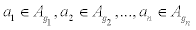and any n-ary operation w ∈ Ω there exists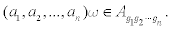We say that a graded Ω-group A is satisfying 1 if the following conditions hold:

1) Described above characteristics are performed;

2) Set of elements (a1, a2,…, an) w are finite for every finite set X ⊆ A if n ≥ 2, a1, a2,…, an ∈ X, w ∈ Ω.

Elements of the set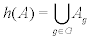are called homogeneous elements of a graded -group A. A nonzero element ag ∈ Ag is called a homogenous element of degree g.

For any nonzero element a ∈ A there exists a unique representation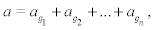where ag ∈ Ag and ag are nonzero elements. The elements i ag are called homogeneous components of a.

Graded Ω-groups can satisfy the conditions that convert them to the group, associative algebra, non-associative algebra, superalgebra, conformal or vertex algebras. Various examples of graded Ω-groups can be found in literature of Balaba .

We can introduce concepts of graded ideal, graded prime radical of graded Ω-groups. We also can consider solvable, nilpotent, locally solvable and locally nilpotent graded Ω-groups. Definitions of these concepts can be found in studies of Balaba .

Finally we shall formulate the analogue of theorem 1 for graded Ω-groups. The proof of this theorem is omitted.

Theorem 2. Let A be a graded Ω-group having the finite condition and satisfying the descending chain condition on graded ideals. Then graded prime radical P(A) of graded Ω-group A is locally nilpotent.

#### References

Select your language of interest to view the total content in your interested language

### Article Usage

• Total views: 8589
• [From(publication date):
December-2015 - Aug 23, 2019]
• Breakdown by view type
• HTML page views : 8399
• PDF downloads : 190

## Post your commentCan't read the image? click here to refresh
###### Peer Reviewed Journals

Make the best use of Scientific Research and information from our 700 + peer reviewed, Open Access Journals

International Conferences 2019-20

Meet Inspiring Speakers and Experts at our 3000+ Global Annual Meetings

Top